# 1 Introduction

The important technological advances we have seen since the publication of the human genome such as microarrays and NGS technologies have allowed us to produce massive amounts of data, making data analysis and interpretation the bottleneck in genomic and epigenomic research. In many cases, this data can be converted to a set of regions in the genome: the positions of the genes in an expression microarray experiment, the regions with peaks in ChIP-seq data or the contact regions in Hi-C. Statistically assessing the spatial relations between these region sets and other region sets or even other genomic features is a fundamental part of this analysis.

regioneR has been created to address this problem and provides functions to statistically evaluate the associations between region sets using permutation tests. Its permutation test framework has been specifically designed to work with genomic regions and all functions are genome- and mask-aware. regioneR includes a number of predefined randomization and evaluation functions covering the most frequent use cases, but the user can also provide custom functions to extend its functionality. In addition to textual output, regioneR also can plot the permutation test results and so it’s possible to go from a set of genomic regions to a publication ready figure in a few lines of code.

In addition, regioneR includes a set of helper functions based on Bioconductor’s GenomicRanges infrastructure to manage and manipulate region sets with a simple and consistent interface.

# 2 Quick Start

One of the most common uses of regioneR is to answer the question: Do the regions in set A overlap with the regions in B more than expected? For example: Are my ChIP-seq peaks on the promoters of active genes? or Are the break points in repetitive regions? To answer question like these we can use the convenience `overlapPermTest` function in regioneR, that evaluates the number of overlaps between two sets of regions.

To do that, we need to have the two region sets either as a `GRanges`, a `data.frame` or a bed-like file. An practical way to create a `GRanges` is to use the `toGRanges` function. In this case, as an example, we will create two sets of random regions with about 50% overlap.

``  A <- createRandomRegions(nregions=50, length.mean=5000000, length.sd=3000000)``
``````##
## Attaching package: 'Biostrings'``````
``````## The following object is masked from 'package:base':
##
##     strsplit``````
``````  B <- c(A[1:25], createRandomRegions(nregions=25, length.mean=500000, length.sd=30000))
numOverlaps(A, B, count.once=TRUE)``````
``##  26``
``  numOverlaps(randomizeRegions(A), B, count.once=TRUE)``
``##  8``

Once we have the two region sets, we can test if A overlaps with B more than expected. To do that we will use `overlapPermTest`.

``  pt <- overlapPermTest(A=A, B=B, ntimes=50)``
``##  "Note: The minimum p-value with only 50 permutations is 0.0196078431372549. You should consider increasing the number of permutations."``
``  pt``
``````## \$numOverlaps
## P-value: 0.0196078431372549
## Z-score: 7.9267
## Number of iterations: 50
## Alternative: greater
## Evaluation of the original region set: 26
## Evaluation function: numOverlaps
## Randomization function: randomizeRegions
##
## attr(,"class")
##  "permTestResultsList"``````

We can see that the test was significant, with a p-value < 0.05. Now, to plot it, we can simply plot the `pt` object.

``  plot(pt)``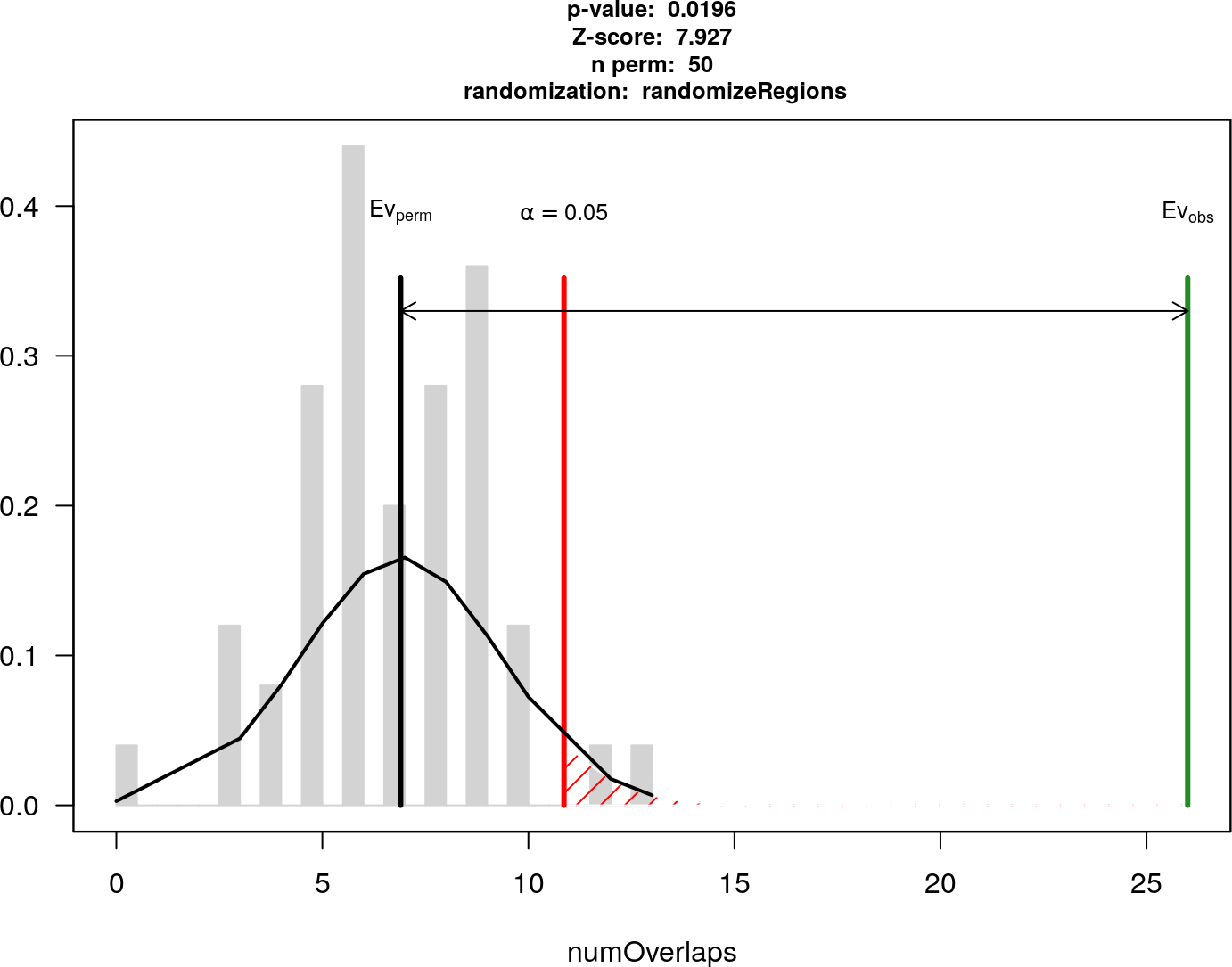We can see a visual representation of the results of the test. In grey the number of overlaps of the randomized regions with B, clustering around the black bar that represents the mean and in green the number of overlaps of the original region set A, which is much larger than expected. The red line denotes the significance limit.

In addition, we can test if the association between the two region sets is highly dependant on their exact position. To do that, we can use the `localZScore` function.

``````  lz <- localZScore(pt=pt, A=A, B=B)
plot(lz)``````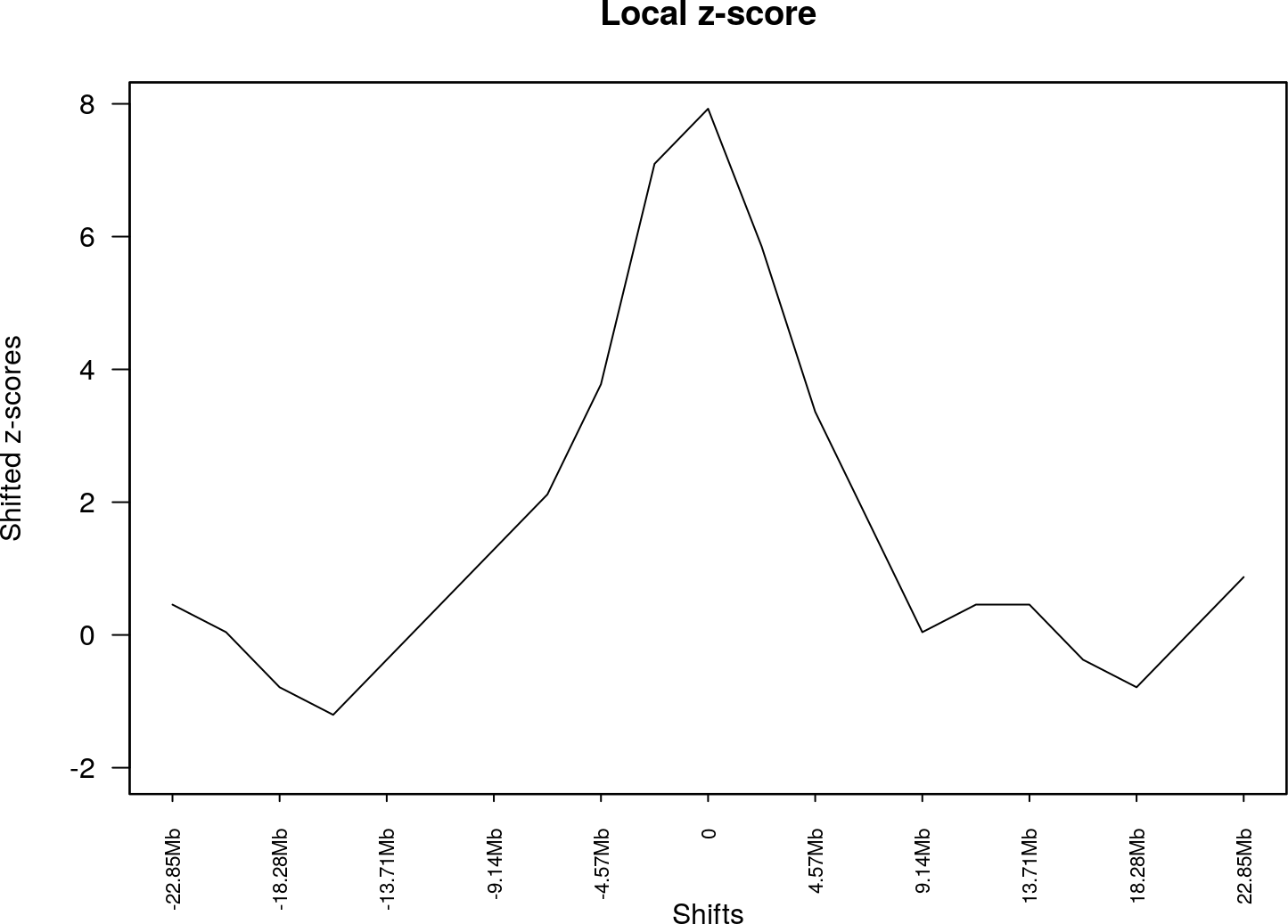Which in this case shows that moving the regions in A produces a drop in the z-scores and so, shows that the association is dependant on the exact position of the regions and is not a regional effect. More information about the `localZScore` function can be found at section Local Z-score.

In this small example we have used `overlapPermTest` to test the number of overlaps between two region sets. Using the more general `permTest` function, we can test other types of association using different evaluation functions (the distance, the evaluation of a function over the genomes or custom user-defined functions) and applying different types of randomizations. In addition, this example assumes that the data refers to the human genome and uses a default mask with regions not available for the randomization. In the next sections, all these options are explained in detail.

# 3 Permutation tests

The core functionality of regioneR is to statistically evaluate the association between different RS or between a RS and other genomic features using a permutation test approach. This functionality is supported by the `permTest` function, a parallel and highly customizable function performing the permutation tests and producing the statistical evaluation of the results.

With `permTest` and the right biological data it is possible to answer different types of biological questions such as:

• Do my regions contain more SNPs than expected by chance?
• Are my regions enriched in repetitive regions?
• Are my regions significantly closer to genes?
• Are DNA methylation levels in my RS higher than expected?
• Do my regions overlap with recurrent SCNAs in my samples?
• Are my ChIP peaks associated with that other histone mark?
• Do my ChIP regions associate with repressed genes?

NOTE: It is important to take into account, however, that regioneR’s permutation tests can only test the association between a set of regions and some other feature but not identify which regions contribute the most to that association. Therefore, for questions of the type “Identify the region in my RS that associate with something”“, regioneR is not a suitable choice, and more specific analysis tools should be used.

## 3.1 How does a permutation test work?

There are 3 main elements needed to perform a permutation test: our RS, a randomization strategy and an evaluation function. Let’s see how it all works with an example:

Imagine we have obtained a set of genes, my special genes, and we want to show they tend to lie in certain parts of the genome, in our case a set of regions we know are altered, for example, they present a copy number gain.

First of all we need to have our RSs loaded into R. To do that we can use the `toGRanges` function.

``````  special <- toGRanges(system.file("extdata", "my.special.genes.txt", package="regioneR"))
all.genes <- toGRanges(system.file("extdata", "all.genes.txt", package="regioneR"))
altered <- toGRanges(system.file("extdata", "my.altered.regions.txt", package="regioneR"))

length(special)``````
``##  200``
``  length(all.genes)``
``##  49646``
``  length(altered)``
``##  8``

Next thing we need is an evaluation function. In our example we want to test the overlap of our RS with the altered regions, so we will use the `numOverlaps` function, which, given two RS returns the number of overlaps between them.

Using `numOverlaps` we can count the number of overlaps between the two RS, in our case we can compute the number of special genes overlapping an altered region.

``  numOverlaps(special, altered)``
``##  114``

But is this number, 114 out of 200, big or small? Does it mean that genes are associated with the altered regions? or might be just by chance?

Here is where the randomization strategy plays its role. We need a randomization strategy that creates a new set of regions that is random with respect to our evaluation function but takes into account the specificities of our original region set. For example, if our original RS comes from an NGS experiment, all of its regions would lie in mappable parts of the genome and wouldn’t make any sense to randomize a region into a centromere or any other non-mappable part of the genome.

To help with that, many randomization functions provided by regioneR accept a mask, indicating where a random region cannot be placed. In any case, selecting the best randomization strategy is a key part of a permutation test and can have a great impact in the final results.

The least restricted function included in regioneR is `randomizeRegions`, that given a RS, a genome and an optional mask, returns a new RS with the same number of regions and of the same width as the original ones but randomly placed along the non-masked parts of the genome. This is also the slowest of the randomization functions available.

In our example, since the special genes are a subset of the bigger set of all genes it is much better to use `resampleRegions`, that given a universe of regions, randomly selects a subset of them to create the randomized region sets.

``````  random.RS <- resampleRegions(special, universe=all.genes)
random.RS``````
``````## GRanges object with 200 ranges and 2 metadata columns:
##         seqnames              ranges strand |           V4          V6
##            <Rle>           <IRanges>  <Rle> |  <character> <character>
##   31045    chr11 124824016-124911385      * |    NM_025004           +
##   42604    chr17   80400462-80408707      * |    NR_036517           -
##   41317    chr17   36337710-36348609      * | NM_001291465           -
##   29800    chr11   58390145-58393205      * |    NM_000614           +
##   33392    chr12 112842993-112847443      * |    NM_000970           -
##     ...      ...                 ...    ... .          ...         ...
##   48795    chr22   46756730-46933067      * |    NM_014246           -
##    6255     chr2         38813-46588      * | NM_001077710           -
##   46506    chr20     9288446-9461462      * |    NM_000933           +
##   14311     chr5 138855112-138862375      * |    NM_198282           -
##   43175    chr18   47015604-47015694      * |    NR_003701           -
##   -------
##   seqinfo: 24 sequences from an unspecified genome; no seqlengths``````

Now, we can use the evaluation function to test the level of association of the randomized RS with the altered regions. And we can repeat that and get different evaluations.

``````  random.RS <- resampleRegions(special, universe=all.genes)
numOverlaps(random.RS, altered)``````
``##  49``
``````  random.RS <- resampleRegions(special, universe=all.genes)
numOverlaps(random.RS, altered)``````
``##  44``
``````  random.RS <- resampleRegions(special, universe=all.genes)
numOverlaps(random.RS, altered)``````
``##  54``
``````  random.RS <- resampleRegions(special, universe=all.genes)
numOverlaps(random.RS, altered)``````
``##  41``

If we do this many times we will build a distribution of the evaluation obtained from random RS and so, we can compare our initial evaluation with those obtained randomly and determine whether it is plausible that our original evaluation was obtained by chance or not. Actually, just counting the number of times the evaluation of the random RS is higher (or lower) than our original evaluation, we can compute the probability of seeing our original evaluation by chance, and that value is exactly the p-value of the permutation test. In addition, we compute the z-score which is the distance between the evaluation of the original RS and the mean of the random evaluations divided by the standard deviation of the random evaluations. The z-score, although not directly comparable, can help in assessing “the strength” of the evaluation.

## 3.2 How to perform a permutation test with regioneR

The main function to perform a permutation test with regioneR is `permTest`, a function taking a a region set (RS) in any of the accepted formats (see section 4 - Region Sets), a randomization function and an evaluation function and returning a `permTestResults` object.

The function performs the whole permutation test analysis described above, evaluating the original RS, creating a number of randomizations and evaluating them and nally computing the p-value and z-score. It takes advantage of the parallel package to randomize and evaluate in parallel where possible.

In addition to the 3 required parameters, `permTest` accepts other fixed parameters -`ntimes` to specify the number of randomizations, `verbose` to toggle the drawing of a progress bar, `force.parallel` to force or forbid the use of multiple cores to run the analysis…- and it also accepts any additional parameter required by the randomization function (usually a genome and a mask) or the evaluation function.

For example to check whether my regions overlap with repeats more than expected, we could use:

``````  # NOT RUN
pt <- permTest(A=my.regions, B=repeats, randomize.function=randomizeRegions,
evaluate.function=numOverlaps)``````

or if we want to check if my special genes have higher methylation levels we could use a test like this:

``````  # NOT RUN
pt <- permTest(A=my.genes, randomize.function=resampleRegions, universe=all.genes,
evaluate.function=meanInRegions, x=methylation.levels.450K)``````

Following our example before with my special genes and the altered regions we could call `permTest` like this:

``````  pt <- permTest(A=special, ntimes=50, randomize.function=resampleRegions, universe=all.genes,
evaluate.function=numOverlaps, B=altered, verbose=FALSE)``````
``##  "Note: The minimum p-value with only 50 permutations is 0.0196078431372549. You should consider increasing the number of permutations."``

NOTE: Since `permTest` uses the ellipsis operator (…) to forward the required additional parameters to the evaluation and randomization functions it is strongly recommended to always use named parameters (e.g. A=RS1 instead of only RS1). Failing to do that can result in hard to debug errors.

In any case we would get a `permTestResults` object with the results of the analysis. To view this result we can just print it or use summary. In this case we can see that the association is statistically significant and so we can conclude that the special genes are associated with my altered regions.

``  pt``
``````## \$numOverlaps
## P-value: 0.0196078431372549
## Z-score: 10.8134
## Number of iterations: 50
## Alternative: greater
## Evaluation of the original region set: 114
## Evaluation function: numOverlaps
## Randomization function: resampleRegions
##
## attr(,"class")
##  "permTestResultsList"``````
``  summary(pt)``
``````## Permutation tests: 1
## Significant permutation tests: 1
## Iterations: 50
## Randomization Function: resampleRegions
## Tests Results:
##                 pvalue  zscore    test
## numOverlaps 0.01960784 10.8134 greater``````

And we can get a graphic representing the results of the permutation test using `plot`. It depicts a gray histogram representing the evaluation of the randomized RS with a fitted normal, a black bar representing the mean of the randomized evaluations and a green bar representing the evaluation of the original RS. In addition, a red bar (and red shading) represents the significance limit (by default 0.05). Thus, if the green bar is in the red shaded region it means that the original evaluation is extremely unlikely and so the p-value will be significant.

``  plot(pt)``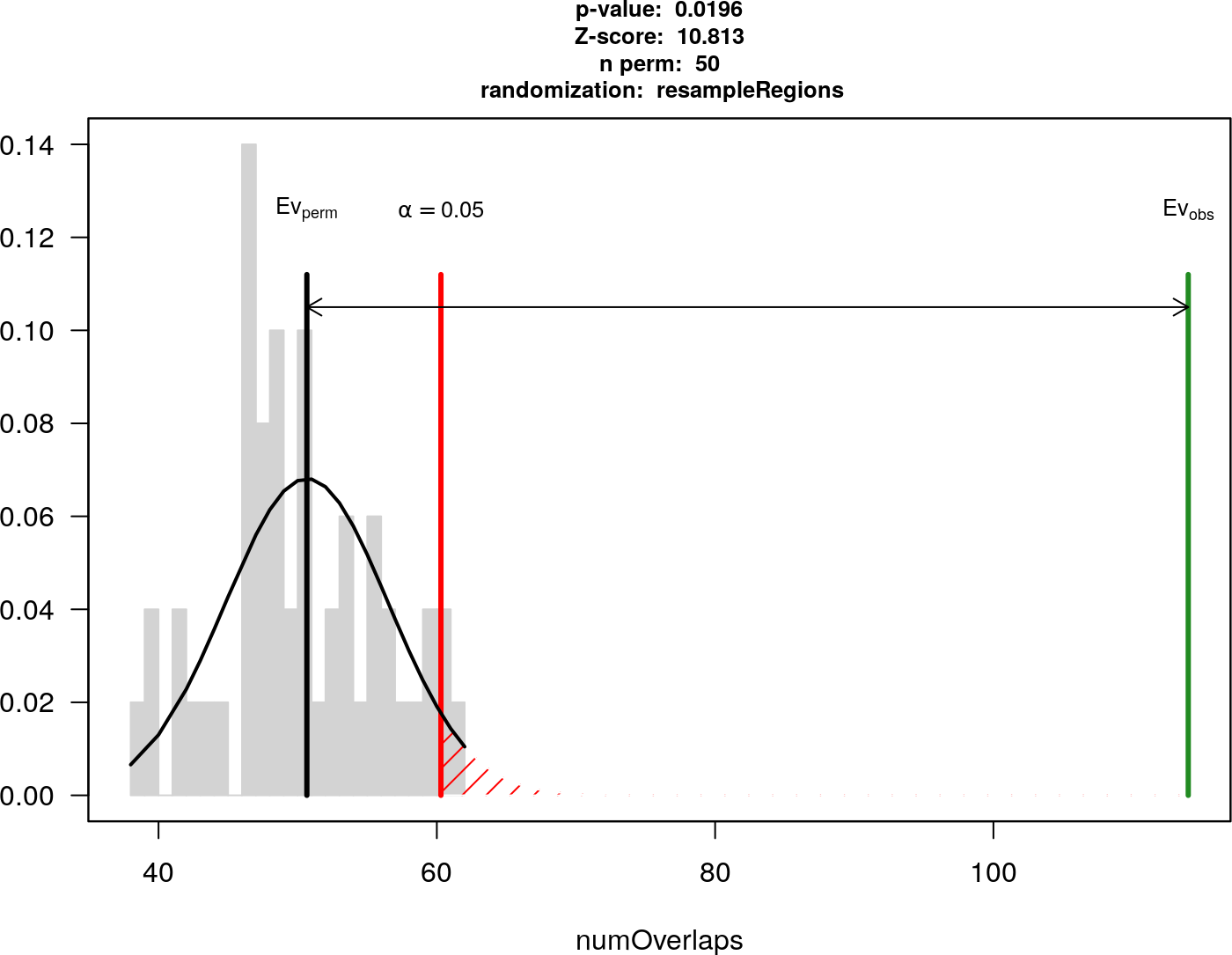To compare, imagine we have a second subset of genes, my regular genes, that are not associated with the altered regions. We can run the same test with them and we will get a negative result. In this case we get a non-significant p-value and we can see in the plot that the original evaluation is close to the mean of the randomized ones.

``````  regular <- toGRanges(system.file("extdata", "my.regular.genes.txt", package="regioneR"))

length(regular)``````
``##  200``
``  numOverlaps(regular, altered)``
``##  46``
``````  pt.reg <- permTest(A=regular, ntimes=50, randomize.function=resampleRegions, universe=all.genes,
evaluate.function=numOverlaps, B=altered, verbose=FALSE)``````
``##  "Note: The minimum p-value with only 50 permutations is 0.0196078431372549. You should consider increasing the number of permutations."``
``  pt.reg``
``````## \$numOverlaps
## P-value: 0.392156862745098
## Z-score: -0.4417
## Number of iterations: 50
## Alternative: less
## Evaluation of the original region set: 46
## Evaluation function: numOverlaps
## Randomization function: resampleRegions
##
## attr(,"class")
##  "permTestResultsList"``````
``  plot(pt.reg)``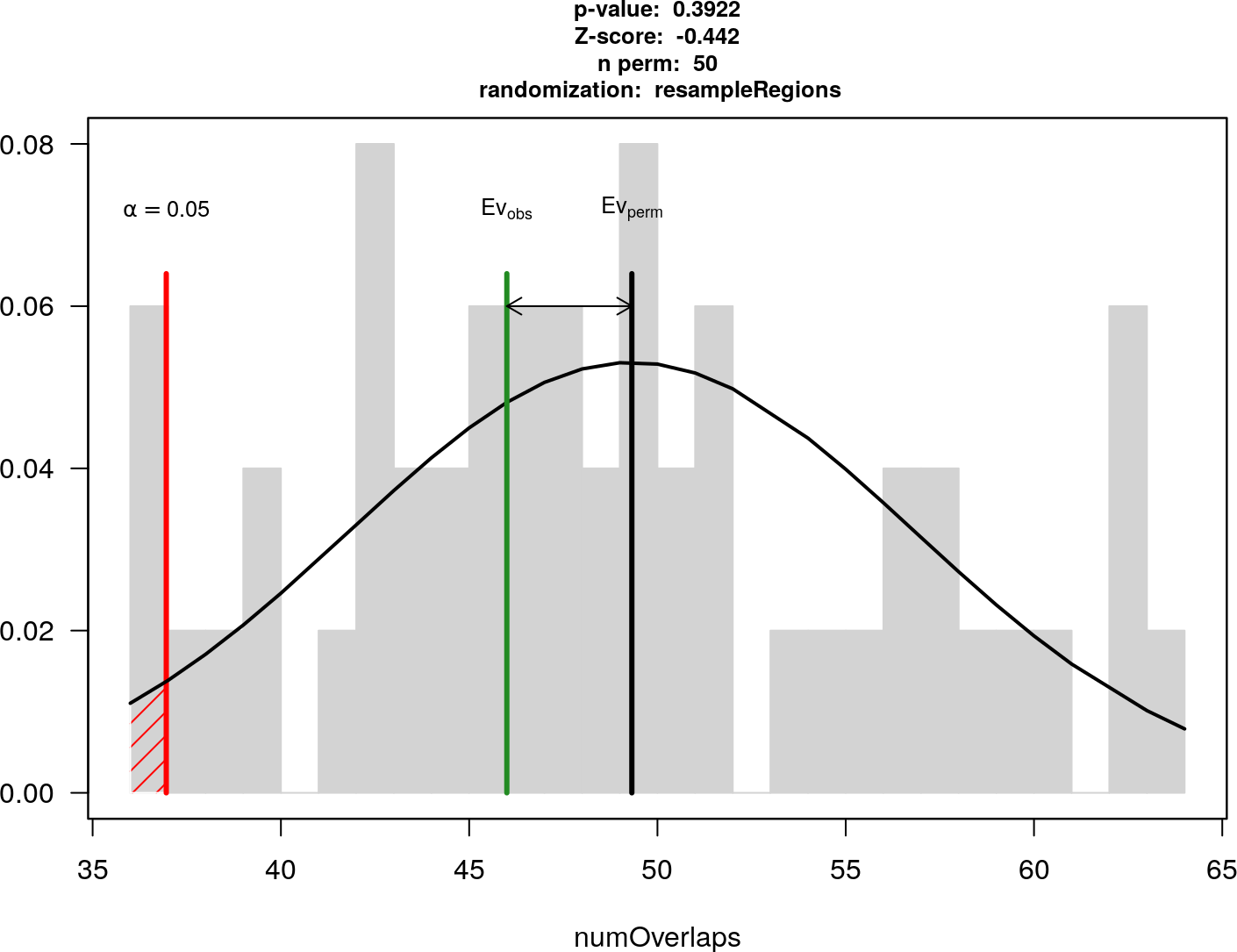## 3.3 A note on the number of permutations

Choosing the right number of permutations is not a simple task. A large number of permutations will produce more accurate results and a nicer-looking plot but a permutation test can be computationally expensive and depending on the number of regions in the RS and the randomization strategy selected it might take up to several hours to perform a permutation test with a few thousand permutations. On the other hand, the lowest p-value is limited by the number of permutations and performing a permutation test with a low permutation number can produce less accurate results. A good strategy could be to try first with a low number of permutations and continue only if the results look promising or at least unclear, since if after some tens of permutations the original evaluation is really close to the mean of the randomizations, the probability it will end up being significant is really small. With a low number of permutations, regioneR will generate a note stating the lowest p-value achievable and ecouraging to increase the permutation number.

As an example, the two permutation tests above, if run with 5000 permutations would produce a plot like these.

``````#NOT RUN - See Figure 1
pt.5000 <- permTest(A=special, ntimes=5000, randomize.function=resampleRegions,
universe=all.genes, evaluate.function=numOverlaps, B=altered, verbose=TRUE)
plot(pt.5000)``````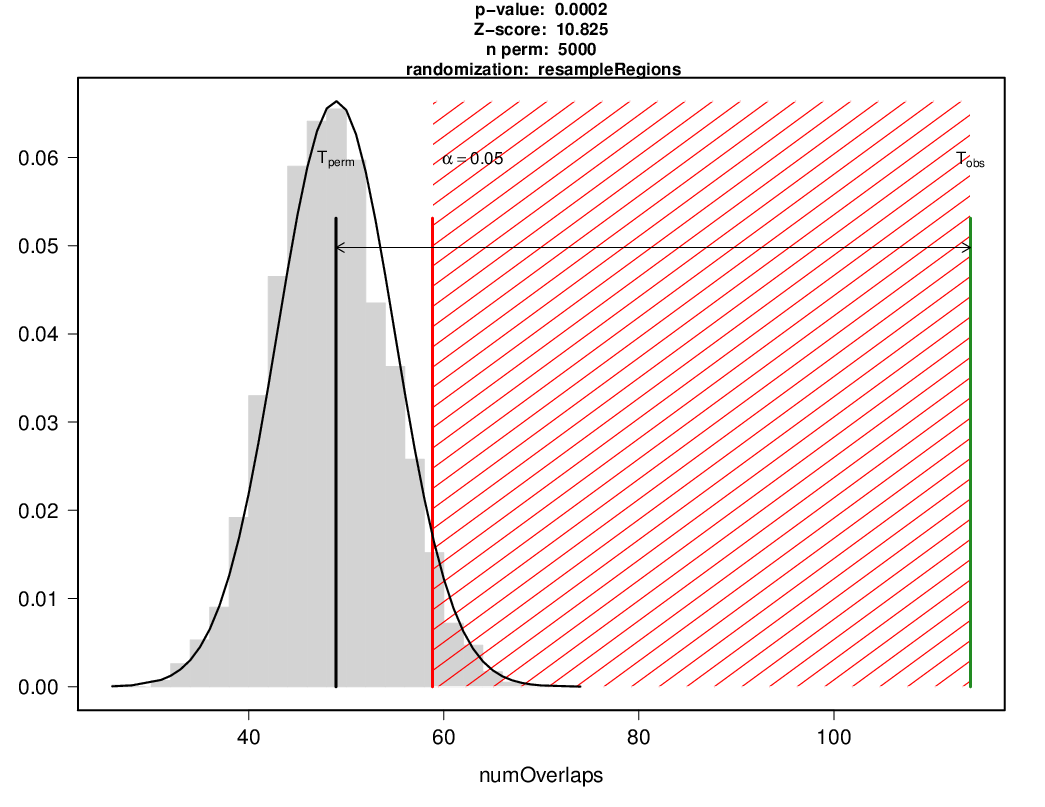This is the plot of a significant permutation test with 5000 permutations.

``````  #NOT RUN - See Figure 2
pt.5000.reg <- permTest(A=regular, ntimes=5000, randomize.function=resampleRegions,
universe=all.genes, evaluate.function=numOverlaps, B=altered, verbose=TRUE)
plot(pt.5000.reg)``````